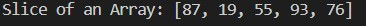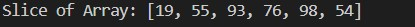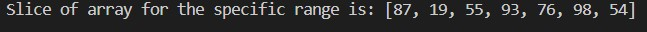# 在 Java 中对数组进行切片

## Java 中的数组切片

``````k[] = {5, 6, 7, 8, 9, 10, 11}
``````

``````k[] = {7, 8, 9, 10}
``````

## 在 Java 中通过复制元素对数组进行切片

``````import java.util.Arrays;
public class Copy {
public static int[] getSlice(int[] arr, int stIndx, int enIndx) {
int[] sclicedArr = new int[enIndx - stIndx];
for (int i = 0; i < sclicedArr.length; i++) {
sclicedArr[i] = arr[stIndx + i];
}
return sclicedArr;
}
public static void main(String args[]) {
int[] arr = { 20, 65, 87, 19, 55, 93, 76, 98, 54, 21 };
int stIndx = 2, enIndx = 6;
int[] sclicedArr = getSlice(arr, stIndx, enIndx + 1);
System.out.println("Slice of an Array: " + Arrays.toString(sclicedArr));
}
}
``````## 使用 Java 中的 `copyOfRange()` 方法对数组进行切片

Java Array 类包含 `copyOfRange()` 方法。它将数组的指定范围复制到新形成的数组（切片数组）中，并返回具有原始数组指定范围的新生成的数组。

``````public static int[] copyOfRange(int[] original, int from, int to)
``````

• `original`：它是一个必须找到切片的数组。
• `from`：它是第一页的索引。它必须在 0 到数组长度的范围内。
• `to`：它是最后一个索引。

``````import java.util.Arrays;
public class Copy1 {
public static int[] slice(int[] arr, int stIndx, int enIndx) {
int[] sclicedArr = Arrays.copyOfRange(arr, stIndx, enIndx);
return sclicedArr;
}
public static void main(String args[]) {
int[] arr = { 20, 65, 87, 19, 55, 93, 76, 98, 54, 21 };
int stIndx = 3, enIndx = 8;
int[] sliceArray = slice(arr, stIndx, enIndx + 1);
System.out.println("Slice of Array: " + Arrays.toString(sliceArray));
}
}
``````## 使用 Java 8 流对数组进行切片

• ##### 打印切片数组。
``````import java.util.Arrays;
import java.util.stream.IntStream;
public class NewClice {
public static int[] findSlice(int[] arr, int stIndx, int enIndx) {
int[] slice_arr = IntStream.range(stIndx, enIndx).map(i -> arr[i]).toArray();
return slice_arr;
}
public static void main(String args[]) {
int[] arr = { 20, 65, 87, 19, 55, 93, 76, 98, 54, 21, 657 };
int stIndx = 2, enIndx = 8;
int[] slice_arr = findSlice(arr, stIndx, enIndx + 1);
System.out.println("Slice of array for the specific range is: " + Arrays.toString(slice_arr));
}
}
``````